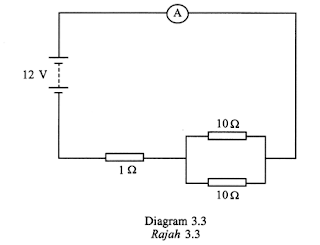Sunday, June 7, 2009

Paper 2 2008 No. 3

3. Diagram 3.1 and Diagram 3.2 show two photographs of electrical circuits.(a) Which diagram shows a parallel circuit?

…………………………………………… [1 mark]

(b) Draw an electrical circuit diagram for the photograph in Diagram 3.1.

[2 marks]

(c) Diagram 3.3 shows an electrical circuit. Assume that the internal resistance of the battery is negligible.(i) Calculate the effective resistance of the circuit.

[2 marks]

(ii) What is the reading of the ammeter?

[1 mark]

(iii) One of the 10 Ω resistors is removed from the circuit. What happens to the ammeter reading?

………………………………………. [1 mark]